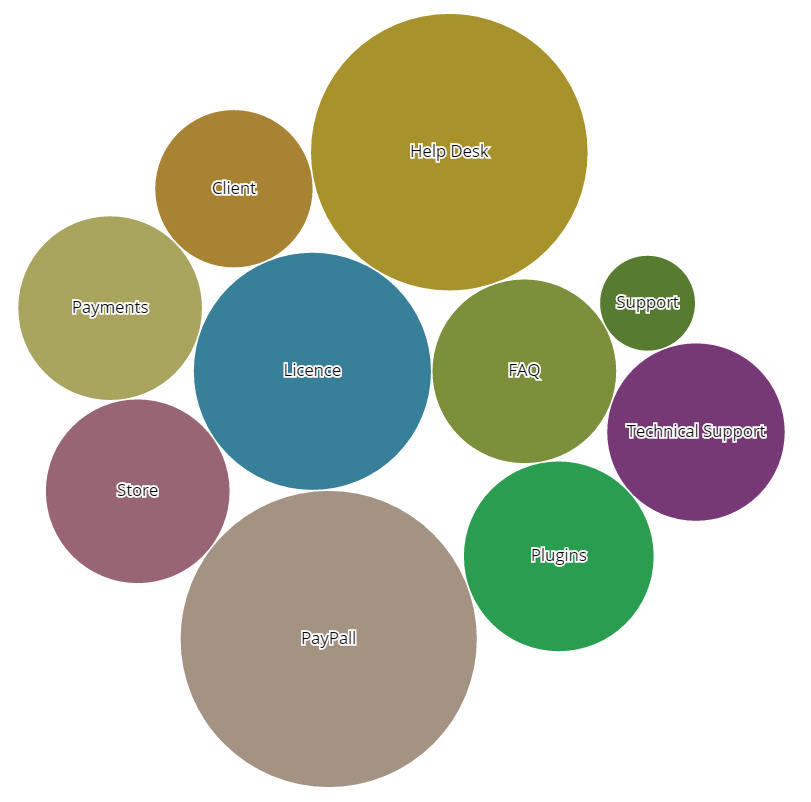# CM Answers Widgets (CMAW) - Shortcodes

## Shortcodes

### TIP: What Are Shortcodes

• Shortcode: [answers-word-cloud width="x" height="y" terms="30" type="cat/tag" size="random/occurrences" minFont="20" maxFont="58"]
• Shortcode attributes:
• width - width of Bubble Word Cloud with unit, for example: width="100%"
• height - height of Bubble Word Cloud with unit, for example: width="300px"
• terms - number of items example: terms="30"
• type - one of two category example: type="tag"
• size - size of font can by random or occurrences, for example: size="random"• Shortcode: [answers-dynamic-cloud width="x" terms="30" type="cat/tag" size="random/occurrences"]
• Shortcode attributes:
• width - width of Bubble Dynamic Cloud example: width="100%"
• terms - number of items example: terms="30"
• type - one of two category example: type="cat"
• size - size of font can by random or occurrences, for example: size="random"• Shortcode: [answers-bubble-graph width="x" terms="30" type="cat/tag" size="random/occurrences"]
• Shortcode attributes:
• width - width of Answers Bubble Graph example: width="100%"
• terms - number of items example: terms="30"
• type - example: type="cat"
• size - size of font can by random or occurrences, for example: size="random"More information about the CM Answers Widgets WordPress  Plugin Other WordPress products can be found at CreativeMinds WordPress StoreLet us know how we can Improve this Product Documentation Page To open a Support Ticket visit our support center